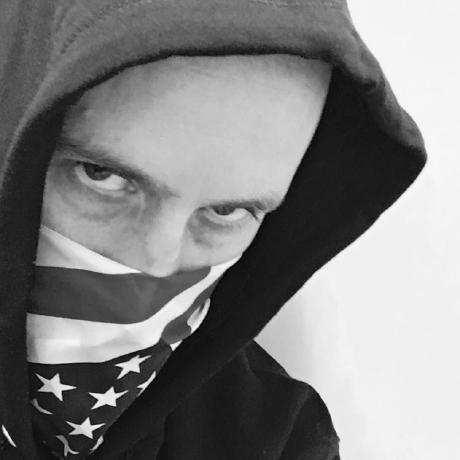# Converting a Decimal Digit to IEEE 754 Binary Floating Point

30 November -0001

IEEE 754 Binary Floating Point is a 32-bit representation (for single precision, 64 bits are used for double precision) for floating point numerals. The 32-bit representation consists of three parts. The first bit is used to indicate if the number is positive or negative. The next 8 bits are used to indicate the exponent of the number, and the last 23 bits are used for the fraction.

The first step in the conversion is the simplest. This is determining the first bit. If the decimal digit is positive then this bit is 0, if the decimal digit is negative then this bit is 1.

The next eight digits are used to express the exponent, which we'll figure out last.

The final 23 digits are used to express the fraction. This gives you 2^23 precision. The first thing to do is convert your decimal digit to binary, ignoring any positive or negative signs. For instance, if your original number was:

```-210.25
```

you first need to convert 210.25 to binary. It is easiest to focus on the integer part of the number first, then the decimals. 210 is 11010010 or 128+64+16+2, otherwise expressed as:

```1*2^7 + 1*2^6 + 0*2^5 + 1*2^4 + 0*2^3 + 0*2^2 + 1*2^1 + 0*2^0
```

Next we need to convert the decimal part. To do this we have to convert the number into a binary sequence that equals a*2-1 + a*2-2 and so on. This series basically means 1/2 + 1/4 + 1/8 + 1/16 and so on. Luckily .25 is 1/4 and so this is easy to calculate. It is .01 or 0*2-1 + 1*2-2. So our final number is 11010010.01. The next step is to normalize this number so that only one non zero decimal place is in the number. To do this you must shift the decimal place 7 positions to the right left. The number 7 becomes important so we note it. This process leaves us with the number 1.101001001 which is the fraction that is represented in the last 23 bit places in the 32 bit binary. However, because of the rules used for conversion the first digit of any number will always be one. Because we know this number there is no need to represent it. Thus the number we will represent is 101001001. This is then padded with 0's to fill in the full 23 bits - leaving us with 10100100100000000000000.

So we now have the first bit, and the last 23 bits of the 32 bit sequence. We must now derive the middle 8 bits. To do this we take our exponent (7) and add 127 (the maximum number you can express with 8 bits (2^8-1 or the numbers 0 to 127)) which gives us 134. We then express this number as an 8 bit binary. This is 10000110 (or 1*2^7 + 1*2^2 + 1*2^1 or 128+4+2). Now we have the middle bits.

Taken as a whole our bit sequence will be:

```1 10000110 10100100100000000000000
```

We can convert this bit sequence back into a number by reversing the process. First we can note by the leading 1 that the number is negative. Next we can determine the exponent. 10000110 is binary for 2+4+128 or 134. 134-127 is 7, so the exponent is 7. Finally we take the last 23 digits, convert them back into the original fraction (adding the preceding 1.) to get:

```1.101001001
```

Moving the decimal place to the right by 7 (corresponding to the exponent) we get:

```11010010.01
```

This binary is equal to 128+64+16+2 + 1/4 or 210.25. Once we apply the negative sign (indicated by the leading bit set to 1) we get our original number:

```-210.25
```

References:

http://en.wikipedia.org/wiki/IEEE_754